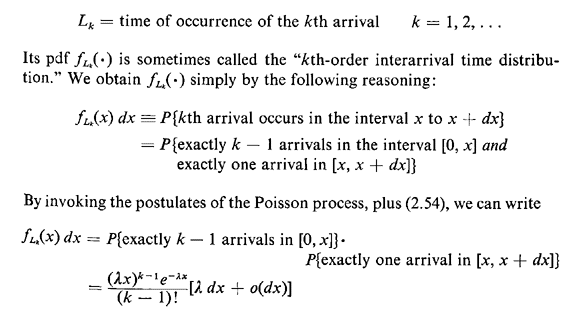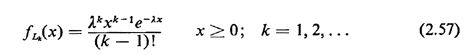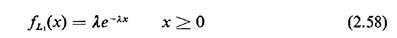## 2.12.2 Interarrival Times

We are now interested in other properties of the Poisson process. For instance, suppose that we start observing the process at time t = 0 and we wish to know the pdf for the time of occurrence of the kth arrival. Define the random variableSince dx is infinitesimal, we can ignore the o(dx) term, and obtain the kth order interarrival time distributionWe have the fundamental result that the kth-order interarrival time distribution for a Poisson process is a kth-order Erlang pdf.

Setting k = 1 in (2.57), we have the first-order arrival distributionThis is our familiar exponential pdf with its now famous "no-memory" property. This result proves that the Poisson process is a no-memory process, that is, that future arrivals do not depend on the number or times of occurrences of previous arrivals.